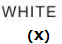>  Test: BITSAT Mock Test- 13

# Test: BITSAT Mock Test- 13

Test Description

## 150 Questions MCQ Test BITSAT Mock Tests Series & Past Year Papers | Test: BITSAT Mock Test- 13

 1 Crore+ students have signed up on EduRev. Have you?
Test: BITSAT Mock Test- 13 - Question 1

### If E₀ represents the peak value of the voltage in an A.C. circuit, the r.m.s. value of the voltage will be

Test: BITSAT Mock Test- 13 - Question 2

### If M is atomic mass, A is mass no. of a nucleus, then M-A/A is called

Test: BITSAT Mock Test- 13 - Question 3

### In a Wheatstone's bridge, all the four arms have equal resistance R. If resistance of the galvanometer arm is also R, then equivalent resistance of the combination is

Detailed Solution for Test: BITSAT Mock Test- 13 - Question 3 The given arrangement is a parallel combination of 2R and 2R. This gives an equivalent resistance of R.
Test: BITSAT Mock Test- 13 - Question 4
According to classical theory, the path of an electron in Rutherford's atom is
Test: BITSAT Mock Test- 13 - Question 5
Two resistances of 400 Ω and 800 Ω are connected in series with 6 volt battery of negligible internal resistance. A voltmeter of resistance 10,000 Ω is used to measure the potential difference across 400 Ω. The error in the measurement of potential difference in volts approximately is
Test: BITSAT Mock Test- 13 - Question 6
Which of the following statements is correct
Test: BITSAT Mock Test- 13 - Question 7

Monochromatic light of frequency 6.0 x 1014 Hz is produced by a laser. The power emitted is 2 x 10 − 3 W . The number of photons emitted, on the average, by the source per second is

Test: BITSAT Mock Test- 13 - Question 8
The resistor R₁ dissipates power P when connected to a generator. If a resistor R₂ is inserted in series with R₁, the power dissipated by R₁
Test: BITSAT Mock Test- 13 - Question 9
The capacity of a condenser 'A' is 10 $\mu$F and it is charged by a battery of 100V. The battery is disconnected and the condenser is connected to a condenser 'B'. The common potential is 40V. The capacity of 'B' is
Test: BITSAT Mock Test- 13 - Question 10
The maximum speed of car on a road turn of radius 30 m; if the coefficient of friction between the tyres and the road is 0.4; will be
Test: BITSAT Mock Test- 13 - Question 11
A satellite of the earth is revolving in a circular orbit with a uniform speed ν. if the gravitational force suddenly disappears, the satellite will
Test: BITSAT Mock Test- 13 - Question 12
According to 'Newton's law of cooling', the rate of cooling of a body is proportional to the
Test: BITSAT Mock Test- 13 - Question 13

A distance 'r' from a point charge, the ratio u/ V2 (where 'u' is energy density and 'V' is potential) is represented by:

Test: BITSAT Mock Test- 13 - Question 14
A polyatomic gas with n degrees of freedom has a mean energy per molecule given by
Test: BITSAT Mock Test- 13 - Question 15
An electron is moving towards east in magnetic field acting vertically downwards. So the electron is deflected towards.
Test: BITSAT Mock Test- 13 - Question 16

Two liquids of densities ρ₁ and ρ₂ and coefficients of viscosity η₁ and η₂ are found to flow through a capillary tube at the same rate. The ratio of η₁/η₂ is

Test: BITSAT Mock Test- 13 - Question 17
The material for which susceptibility remains constant for small values of magnetising field, increases for large values and then decreases for very large values is
Test: BITSAT Mock Test- 13 - Question 18
In a capillary tube, water rises upto 3 mm. The height of water that will rise in another capillary tube having one-third radius of the first is
Test: BITSAT Mock Test- 13 - Question 19

A wire of length L and area of cross-section A is made of material of Young's modulus y. If the wire is stretched by the amount x, the work done is

Test: BITSAT Mock Test- 13 - Question 20
A body starts to fall freely under gravity. The distances covered by it in first, second and third second are in ratio
Test: BITSAT Mock Test- 13 - Question 21
Which of the following cannot be resultant of the vectors of magnitude 5 and 10?
Test: BITSAT Mock Test- 13 - Question 22

A projectile can have the same range 'R' for two angles of projection. If 't₁' and 't₂' be the times of flights in the two cases, then the product of the two time of flights is proportional to

Test: BITSAT Mock Test- 13 - Question 23

A block of mass 'm' rests on a horizontal table. A string tied to the block is passed on a frictionless pulley fixed at the end of the table and to the other end of string is hung another block of mass 'm'2. The acceleration of the system is

Test: BITSAT Mock Test- 13 - Question 24

The kinetic energy of a particle, executing simple harmonic motion is 16 J when it is in its mean position. If the amplitude of oscillations is 25 cm and the mass of the particles is 5.12 kg, the time period of its oscillation is

Detailed Solution for Test: BITSAT Mock Test- 13 - Question 24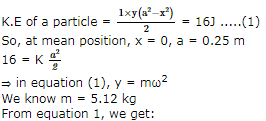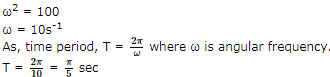Test: BITSAT Mock Test- 13 - Question 25
In an astronomical telescope, the focal lengths of objective lens and eye-piece are 180 cm and 6 cm respectively. In normal adjustment, magnifying power of the astronomical telescope will be
Test: BITSAT Mock Test- 13 - Question 26

Two points are located at a distance of 10 m and 15 m from the source of oscillation . The period of oscillation is 0.05 sec and the velocity of the wave is 300 m/sec. What is the phase difference between the oscillations of two points ?

Test: BITSAT Mock Test- 13 - Question 27
Wave lengths of light used in an optical instrument are λ = 4000 Å and λ = 5000 Å. The ratio of their respective resolving power is
Test: BITSAT Mock Test- 13 - Question 28
A circular turn table has a block of ice placed at its centre. The system rotates with an angular speed ω about an axis passing through the centre of the table. If the ice melts on its own without any evaporation, the speed of rotation of the system
Test: BITSAT Mock Test- 13 - Question 29
Which of these statements is not correct ?
Test: BITSAT Mock Test- 13 - Question 30

If I is the moment of inertia of a body and ω is its angular velocity, then the rotational kinetic energy is

Test: BITSAT Mock Test- 13 - Question 31

The neutral temperature of a thermocouple is 350oC when the cold junction is at 0oC.When the cold junction is immersed in a bath of 30oC,the inversion temperature is

Test: BITSAT Mock Test- 13 - Question 32
A container that suits the occurrence of an isothermal process should be made of
Test: BITSAT Mock Test- 13 - Question 33
Who gave the universal law of gravitation?
Test: BITSAT Mock Test- 13 - Question 34

A gas is compressed at a constant pressure of 50 N/m2 from a volume of 10 m3 to a volume of 4 m3. Energy of 100 J is then added to the gas by heating. Its internal energy is

Test: BITSAT Mock Test- 13 - Question 35

In Young's double slit experiment, the width of the fringes obtained with light of wavelength 6000 Angstrom is 2.0mm. The fringe width, if the entire apparatus is immersed in a liquid of refractive index 1.33, will be

Detailed Solution for Test: BITSAT Mock Test- 13 - Question 35

Fringe width in Young's double slit experiment β = Dλ /d
When apparatus is immersed in liquid, only wavelength of light (λ) changes
Wavelength of light is liquid,
λ' = λ / n , where n = refractive index of medium
Initial fringe width, β = Dλ /d .....(i)
Fringe width in liquid, β' = Dλ' /d .....(ii)
Dividing Equation (ii) by Equation (i), we get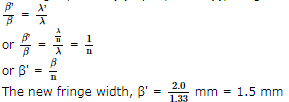Test: BITSAT Mock Test- 13 - Question 36
The sun is rotating about is own axis. The spectral lines emitted from the two ends of its equator, for an observer on the earth, will show
Test: BITSAT Mock Test- 13 - Question 37
The speed of sound in air at a given temperature is 350 m/s. An engine blows whistle at a frequency of 1200 c/s. It is approaching the observer with velocity 50 m/s. The apparent frequency in c/s heard by the observer will be
Test: BITSAT Mock Test- 13 - Question 38

The phase difference between two points separated by 0.8 m is π/4. If velocity of the wave is 256 m-s⁻1, then its frequency will be

Test: BITSAT Mock Test- 13 - Question 39
A ball moves in a frictionless inclined table without slipping. The work done by the table surface on the ball is
Test: BITSAT Mock Test- 13 - Question 40
A spriong 40 mm long is stretched by the application of a force. If 10 N force required to stretch the spring through 1 mm, then work done in stretching the spring through 40 mm is
Test: BITSAT Mock Test- 13 - Question 41
Acetyl salicylic acid is used as
Test: BITSAT Mock Test- 13 - Question 42
Reduction of a carbonyl group to a methylene group by alkaline decomposition of the hydrazone is known as
Test: BITSAT Mock Test- 13 - Question 43
Who modified Bohr's theory by introducing elliptical orbits for electron path?
Test: BITSAT Mock Test- 13 - Question 44
The ratio of specific charge of a proton and an α-particle is
Test: BITSAT Mock Test- 13 - Question 45
Heterolytic fission of a covalent bond in organic molecules gives
Test: BITSAT Mock Test- 13 - Question 46
Vapour density of a gas is 22. What is its molecular mass?
Test: BITSAT Mock Test- 13 - Question 47
In the process of nitration, the electrophile formed is
Test: BITSAT Mock Test- 13 - Question 48
Which of the following is an electrophile?
Test: BITSAT Mock Test- 13 - Question 49
Test: BITSAT Mock Test- 13 - Question 50
Cholesterol is
Test: BITSAT Mock Test- 13 - Question 51
Dimerisation in carboxylic acid is due to
Test: BITSAT Mock Test- 13 - Question 52

Which of the following is not isostructural with SiCl₄?

Test: BITSAT Mock Test- 13 - Question 53
The state of equilibrium refers to
Test: BITSAT Mock Test- 13 - Question 54
Which of the following does not represent the criterion of spontaneity ?
Test: BITSAT Mock Test- 13 - Question 55

The rate constant for a first order reaction whose half life is 480 sec. is

Test: BITSAT Mock Test- 13 - Question 56
The I.P. of an element on going from left to right in a period
Detailed Solution for Test: BITSAT Mock Test- 13 - Question 56 As we move from left to right in a period, the ionisation potential increases with increasing atomic numbers. This is because across a period the nuclear charge increases though the principal quamtum number of the valence shell remains the same. As a result, the valence electrons are more and more tightly held by the nucleus. Consequently, more energy is needed to remove the electrons and hence ionisation potential keeps on increasing.
Test: BITSAT Mock Test- 13 - Question 57

The amount of heat required to raise the temperature of a body temperature through 1oC is called, its

Test: BITSAT Mock Test- 13 - Question 58
Which of the following gas is used in anaesthesia?
Test: BITSAT Mock Test- 13 - Question 59

Which of the following complex ion is paramagnetic?

Test: BITSAT Mock Test- 13 - Question 60
Potassium dichromate is obtained from which of the following ore?
Test: BITSAT Mock Test- 13 - Question 61
Lanthanoids are 14 elements in the
Test: BITSAT Mock Test- 13 - Question 62
The chemical reaction taking place at anode is
Test: BITSAT Mock Test- 13 - Question 63
A carbon-carbon double bond consists of
Test: BITSAT Mock Test- 13 - Question 64
Which of the following is not a meta-directing group?
Detailed Solution for Test: BITSAT Mock Test- 13 - Question 64 The substituents or groups which direct the incoming group to ortho and para positions are called ortho, para-directing groups.
For example, -CH3, -NH2, -NHCH3 etc.
The substituents or groups which direct the incoming group to the metaposition are called meta- directing groups. For example,
-NO2, -CN, -SO3H etc.
So the correct answer is -NH2
Test: BITSAT Mock Test- 13 - Question 65
Hydrogen directly combines with
Test: BITSAT Mock Test- 13 - Question 66
The concept that acid is proton donor and base is proton acceptor was given by
Test: BITSAT Mock Test- 13 - Question 67
A similarity between optical and geometrical isomerism is that
Test: BITSAT Mock Test- 13 - Question 68
An alkyl halide reacts with metallic sodium in dry ether the reaction is known as
Test: BITSAT Mock Test- 13 - Question 69
Which of the following leaves no residue on heating?
Test: BITSAT Mock Test- 13 - Question 70
The shape of carbon dioxide molecule is
Test: BITSAT Mock Test- 13 - Question 71
The first ionisation potential of Group 13 elements is lower than that of Group 2 elements, because
Test: BITSAT Mock Test- 13 - Question 72
Synthetic polymer prepared from caprolactum is known as
Test: BITSAT Mock Test- 13 - Question 73
Which of the following statement is correct with respect to the property of elements with increase in atomic no. in the carbon family?
Test: BITSAT Mock Test- 13 - Question 74

Which of the following is correct about the reaction?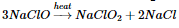Test: BITSAT Mock Test- 13 - Question 75
Which of the following pairs liberates a gas when they react with each other?
Test: BITSAT Mock Test- 13 - Question 76
Which one of the following electrolytes are used in Down's process of extracting sodium metal
Test: BITSAT Mock Test- 13 - Question 77
The relative lowering of the vapour pressure is equal to the ratio between the number of
Test: BITSAT Mock Test- 13 - Question 78
A pure crystalline substance on being heated gradually first forms a turbid liquid at constant temperature and still at a higher temperature, the turbidity completely disappears. The behaviour is a characteristic of substance forming
Test: BITSAT Mock Test- 13 - Question 79
Which of the following is used for the destruction of colloids?
Test: BITSAT Mock Test- 13 - Question 80

The area in square units bounded by the curves y = x3, y = x2 and the ordinates x = 1, x = 2 is

Test: BITSAT Mock Test- 13 - Question 81

The molecular mass of CO2 is 44 a.m.u. Avogadro Number is 6.02 x 1023 . Therefore the mass of one molecule of CO2 is

Test: BITSAT Mock Test- 13 - Question 82

In the expansion of (1+x)n (1-x)m the coefficient of x and x2 are 3 and -6 respectively, then m is

Test: BITSAT Mock Test- 13 - Question 83

The limiting point of the system of co-axial circles x2+y2-6x-6y+4=0, x2+y2-2x-4y+3=0 is

Test: BITSAT Mock Test- 13 - Question 84

Length of the common chord of the circles x2 + y2 + 5x + 7y + 9 = 0 and x2 + y2 + 7x + 5y + 9 = 0 is

Test: BITSAT Mock Test- 13 - Question 85
If -1 + √-3 = reiiθ, then θ =
Test: BITSAT Mock Test- 13 - Question 86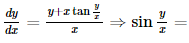Test: BITSAT Mock Test- 13 - Question 87

The degree of the differential equation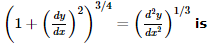Detailed Solution for Test: BITSAT Mock Test- 13 - Question 87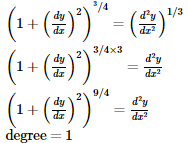Test: BITSAT Mock Test- 13 - Question 88
Solution of cos x dy/dx+y sin x =1 is
Test: BITSAT Mock Test- 13 - Question 89
What is the derivative of f(x) = x|x| ?
Test: BITSAT Mock Test- 13 - Question 90

In which interval the function f(x) = - 2x3 - 9x2 - 12x + 1 is decreasing

Detailed Solution for Test: BITSAT Mock Test- 13 - Question 90

f(x) = - 2x3 - 9x2 - 12x + 1
f' (x) = - 6x2 - 18x - 12
Since f(x) is decreasing
∴ f'(x) < 0
⇒ - 6x2 - 18x - 12 < 0
or x2 + 3x + 2 > 0
or (x + 1) (x + 2) > 0
∴ The interval is (-∞, -2) and (-1, ∞)

Test: BITSAT Mock Test- 13 - Question 91

2/1! + 4/3! + 6/5! + .........∞ is equal to

Test: BITSAT Mock Test- 13 - Question 92

If two towers of heights h₁ and h₂ subtend angles 60o and 30o respectively at the midpoint of the line joining their feet, then h₁:h₂

Test: BITSAT Mock Test- 13 - Question 93

If the two intersecting lines intersect the hyperbola and neither of them is a tangent to it, then number of intersecting points are

Detailed Solution for Test: BITSAT Mock Test- 13 - Question 93

The general equation of a straight line is linear: ax + by + c = and the general equation of a hyperbola (but it's the same for all conics) is a second degree equation:
1. Ax2 + Bxy + Cy2 + Dx + Ey + F = 0
So, finding y ( or x ) from the first equation and substituting in the second, we have a second degree equation that has, at most, two real solutions. The possible cases are:
1) two real distinct solutions: the line is secant at two points
2) two coincident solution: the line is tangent
3) no real solution : the line is e''external''
4) the equation reduce to a first degree equation so we have one real solution and we say that the other solution goes to infinity.
So, for two lines that are not tangent we can have:
no common point with the conic if the two lines are external,
2 points if one is secant at two pints and the other is external or if both have one real common point with the conic an the other at infinity,
3 common points if one is secant at two points and the other at one point,
4 common points if the two lines are both secants at two points.

Test: BITSAT Mock Test- 13 - Question 94

A primitive of |x|, when x < 0 is

Test: BITSAT Mock Test- 13 - Question 95

Which of the following functions is a solution of the differential equation (dy/dx)2 - x (dy/dx) + y = 0?

Test: BITSAT Mock Test- 13 - Question 96

sec⁻1[sec(-30o)]=

Test: BITSAT Mock Test- 13 - Question 97
The set of all points, where the function
f(x) = [x/(1 + ∣x∣)] is differentiable, is
Test: BITSAT Mock Test- 13 - Question 98

For all integers n ≥ 1 , which of the following is divisible by 9

Detailed Solution for Test: BITSAT Mock Test- 13 - Question 98

If n = 2 then 8n + 1 = 65, 32n + 3n + 1 = 88, 10n + 1 = 101 which are not divisible by 9
But 4n - 3n - 1 = 9, divisible by 9

Test: BITSAT Mock Test- 13 - Question 99
If in a square matrix A=[aij], we find that
aij = aji ∀ i,j, then A is a
Test: BITSAT Mock Test- 13 - Question 100

The point on the curve y = x2 + 4x + 3 which is closest to the line y = 3x + 2 is

Test: BITSAT Mock Test- 13 - Question 101

If A and B are square matrices and A⁻1 and B⁻1 of same order exists, then (AB)⁻1 is equal to

Test: BITSAT Mock Test- 13 - Question 102
If √3 + i = (a+ib) (c+id), then tan -1b/a + tan -1 d/c is equal to
Test: BITSAT Mock Test- 13 - Question 103

The equation of pair of lines which are perpendicular to the pair of lines ax2+2hxy+by2=0 is

Test: BITSAT Mock Test- 13 - Question 104

If the normal at the point t on a parabola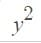= 4ax meet it again at t2, then t2 =

Detailed Solution for Test: BITSAT Mock Test- 13 - Question 104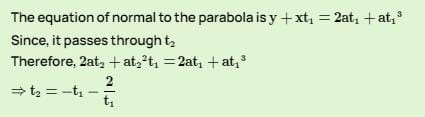Test: BITSAT Mock Test- 13 - Question 105

For the parabola y2 + 6 y − 2 x + 5 = 0
1. The vertex is − 2, − 3
2. The directrix is y + 3 = 0
Which of the following is correct?

Test: BITSAT Mock Test- 13 - Question 106
How many total words can be formed from the letters of the word INSURANCE in which vowels are always together?
Test: BITSAT Mock Test- 13 - Question 107
How many nine digit numbers can be formed using the digits 2, 2, 3, 3, 5, 5, 8, 8, 8 so that the odd digits occupy even positions?
Test: BITSAT Mock Test- 13 - Question 108
For a B.D., the parameters n and p are 16 and 1/2 respectively. Then its S.D.σ is equal to
Test: BITSAT Mock Test- 13 - Question 109
In eight throws of 1 die or 3 is considered a success. Then the standard deviation of the success is
Test: BITSAT Mock Test- 13 - Question 110

The angles of a triangle are in the ratio 3:5:10. Then the ratio of the smallest side to the greatest side is

Test: BITSAT Mock Test- 13 - Question 111

In Δ A B C , If a2 + b2 + c2 = 8R2 , then the triangle is

Detailed Solution for Test: BITSAT Mock Test- 13 - Question 111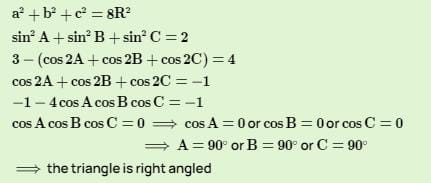Test: BITSAT Mock Test- 13 - Question 112

If a, b and c are real numbers such that a2 + b2 + c2 = 1, then ab + bc + ca lies in the interval

Test: BITSAT Mock Test- 13 - Question 113
For a series whose nth term is (n/x) + (y), the sum of r terms is
Test: BITSAT Mock Test- 13 - Question 114

If the pth, qth and rth terms of a G.P. are l, m and n respectively, then lq-r mr-p np-q is

Detailed Solution for Test: BITSAT Mock Test- 13 - Question 114

Let A and R be the first term and common ratio of G.P. respectively
nth term of G.P., ln = ARn - 1
pth term of G.P. = l
∴ ARp - 1 = l.....(1)
qth term of G.P. = m
∴ ARq - 1 = m .....(2)
rth term of G.P. = n
∴ ARr - 1 = n.....(3)
lq - r mr - p np - q
= (ARp - 1)q - r * (ARq - 1)r - p * (ARr - 1)p - q
= Aq - r R(p - 1) (q - r) * Ar - p R(q - 1) (r - p) * Ap - q R(r - 1) (p - q)
= Aq - r + r - p + p - q * Rpq - pr - q + r + qr - pq - r + p + pr - qr - p + q
= A0 * R0
= 1 * 1
= 1

Test: BITSAT Mock Test- 13 - Question 115

If f : N x N → N is such that f (m,n) = m + n where N is the set of natural numbers, then which of the following is true?

Test: BITSAT Mock Test- 13 - Question 116

If f(x) = (2x - 2-x)/(2x + 2-x), then f⁻1(x) is

Test: BITSAT Mock Test- 13 - Question 117
The equation of line passing through (3,2) and perpendicular to y=x is
Test: BITSAT Mock Test- 13 - Question 118
The tangent to the curve y=e2x at the point (0,1) meets the x-axis at
Test: BITSAT Mock Test- 13 - Question 119

The equation of the sphere with centre (3, 6, -4) and touching the plane
2x - 2y - z - 10 = 0 is

Detailed Solution for Test: BITSAT Mock Test- 13 - Question 119

As the required sphere touches the plane
2x - 2y - z - 10 = 0 .....(1)
∴ Radius of the required sphere = ⊥ from the center (3, 6, -4) of the required sphere to the plane (1)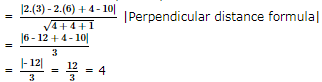∴ The required equation of the sphere is given by
(x - 3)2 + (y - 6)2 + (z + 4)2 = 16 (Central form)
⇒ x2 + 9 - 6x + y2 + 36 - 12y + z2 + 16 + 8z = 16
⇒ x2 + y2 + z2 - 6x - 12y + 8z + 45 = 0

Test: BITSAT Mock Test- 13 - Question 120
The plane XOZ divides the join of (1, -1, 5) and (2, 3, 4) in the ratio λ : 1, then λ is
Test: BITSAT Mock Test- 13 - Question 121

If tan(πcosθ)=cot(πsinθ), then cos[(θ)-(π/4)]=

Test: BITSAT Mock Test- 13 - Question 122

The general solution of sin2 x − 2 cos x + 1/4 = 0 is

Test: BITSAT Mock Test- 13 - Question 123

sin 50o-sin 70o+sin 10o is equal to

Test: BITSAT Mock Test- 13 - Question 124
If i,j,k are unit and mutually perpendicular vectors, [ikj]=
Test: BITSAT Mock Test- 13 - Question 125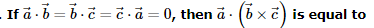Test: BITSAT Mock Test- 13 - Question 126
Find the Antonym of the word occurring in the sentence in capital letter as per the context.
All these measures will AUGMENT employment.
Detailed Solution for Test: BITSAT Mock Test- 13 - Question 126 AUGMENT means 'to increase the value, size, amount etc'
DIMINISH means 'to become or make something become smaller, weaker etc'
Test: BITSAT Mock Test- 13 - Question 127
Fill in the blank with appropriate word.
They are refugees in need of........
Test: BITSAT Mock Test- 13 - Question 128
Fill in the blank with appropriate word.
The most important task of the Air Force is to.........the country against an air attack by an enemy.
Test: BITSAT Mock Test- 13 - Question 129
Find the Antonym of the word occurring in the sentence in capital letter as per the context.
I have MALICE towards none.
Detailed Solution for Test: BITSAT Mock Test- 13 - Question 129 'Malice' means feeling of hatred.
Its antonym will be 'Sympathy'
Test: BITSAT Mock Test- 13 - Question 130
Choose the one which can be substituted for the given words/sentences :
One who can use either of his hands with ease
Test: BITSAT Mock Test- 13 - Question 131
Spot the error.
Test: BITSAT Mock Test- 13 - Question 132
Choose the one which can be substituted for the given words/sentences
To walk with slow or regular steps is to
Test: BITSAT Mock Test- 13 - Question 133
Find the Synonym of the word occurring in the sentence in capital letter as per the context.
This job is very TEDIOUS.
Detailed Solution for Test: BITSAT Mock Test- 13 - Question 133 Meaning of TEDIOUS - Tiresome by reason of length, slowness, or dullness; boring
Tiresome is direct link as being a boring job can be a reason of tiring but it is not the direct meaning of the word
Test: BITSAT Mock Test- 13 - Question 134
Spot the error.
Detailed Solution for Test: BITSAT Mock Test- 13 - Question 134 the train had left
Test: BITSAT Mock Test- 13 - Question 135
Find the Synonym of the word occurring in the sentence in capital letter as per the context.
The spectators looked at the batsman in AMAZEMENT when he hit sixer after sixer.
Detailed Solution for Test: BITSAT Mock Test- 13 - Question 135 Meaning of AMAZEMENT - A state of extreme surprise or wonder; astonishment
In the context of situation given in sentence it can be said that people will look batsman in wonder but not in surprise after titting a sixer as batsman is there to score maximu, there is not surprise if he hits a sixer
But there can be a wonderful feeling in spectators
Test: BITSAT Mock Test- 13 - Question 136
Spot the error.
Test: BITSAT Mock Test- 13 - Question 137
Choose the word which is least like the other words in the group .
Test: BITSAT Mock Test- 13 - Question 138
Complete the analogous pair.
Car : Petrol : : Television : ?
Test: BITSAT Mock Test- 13 - Question 139
Find out the term in the number series which is wrong.
1,5,11,19,29,55
Detailed Solution for Test: BITSAT Mock Test- 13 - Question 139 5 - 1 = 4; 11 - 5 = 6; 19 - 11 = 8
29 - 19 = 10; 55 - 29 = 26
Pattern gets disturbed at last stage
∴ 55 is wrong
It should be 29 + 12 = 41
Test: BITSAT Mock Test- 13 - Question 140
In this letter series, some of the letters are missing. Choose the correct letter given below -
_ baa _ aab _ a _ a
Test: BITSAT Mock Test- 13 - Question 141

Group the following figures into three classes on the basis of identical properties.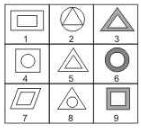Detailed Solution for Test: BITSAT Mock Test- 13 - Question 141

1,5,7 have two similar elements, one inside the other.
2,4,8 have one element placed inside a different element.
3,6,9 have two similar elements, one inside the ohter and the area between the two elements is shaded.

Test: BITSAT Mock Test- 13 - Question 142

Find the missing character.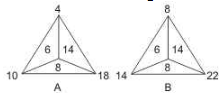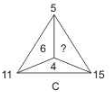Detailed Solution for Test: BITSAT Mock Test- 13 - Question 142

In fig. (A), 10 - 4 = 6, 18 - 10 = 8, 18 - 4 = 14.
In fig. (B), 14 - 8 = 6, 22 - 14 = 8, 22 - 8 = 14.
In fig. (C), 11 - 5 = 6, 15 - 11 = 4.
So, missing number = 15 - 5 = 10.

Test: BITSAT Mock Test- 13 - Question 143

Diversity typically provides fresh perspectives on issues, but it makes it more difficult to unify the team and reach agreements. The strongest case for diversity on work teams is when these teams are engaged in problem-solving and decision-making tasks. Heterogeneous teams bring multiple perspectives to the discussion, thus increasing the likelihood that the team will identify creative or unique solutions. Additionally, the lack of common perspective usually means diverse teams spend more time discussing issues, which decrease the chances that a weak alternative will be chosen. However, keep in mind that the positive contribution that diversity makes to decision-making teams undoubtedly declines over time. Diverse groups have more difficulty working together and solving problems, but this dissipates with time. Expect the value- added component of diverse teams to decrease as members become more familiar with each other and the team becomes more cohesive.

Q. Diversity on work team leads to

Test: BITSAT Mock Test- 13 - Question 144

Diversity typically provides fresh perspectives on issues, but it makes it more difficult to unify the team and reach agreements. The strongest case for diversity on work teams is when these teams are engaged in problem-solving and decision-making tasks. Heterogeneous teams bring multiple perspectives to the discussion, thus increasing the likelihood that the team will identify creative or unique solutions. Additionally, the lack of common perspective usually means diverse teams spend more time discussing issues, which decrease the chances that a weak alternative will be chosen. However, keep in mind that the positive contribution that diversity makes to decision-making teams undoubtedly declines over time. Diverse groups have more difficulty working together and solving problems, but this dissipates with time. Expect the value- added component of diverse teams to decrease as members become more familiar with each other and the team becomes more cohesive.

Q. With diversity in work-force there is

Test: BITSAT Mock Test- 13 - Question 145

Diversity typically provides fresh perspectives on issues, but it makes it more difficult to unify the team and reach agreements. The strongest case for diversity on work teams is when these teams are engaged in problem-solving and decision-making tasks. Heterogeneous teams bring multiple perspectives to the discussion, thus increasing the likelihood that the team will identify creative or unique solutions. Additionally, the lack of common perspective usually means diverse teams spend more time discussing issues, which decrease the chances that a weak alternative will be chosen. However, keep in mind that the positive contribution that diversity makes to decision-making teams undoubtedly declines over time. Diverse groups have more difficulty working together and solving problems, but this dissipates with time. Expect the value- added component of diverse teams to decrease as members become more familiar with each other and the team becomes more cohesive.

Q.